Function Repository Resource:

# GaussianCurvature

Compute the Gaussian curvature of a surface

Contributed by: Alfred Gray
 ResourceFunction["GaussianCurvature"][s,{u,v}] computes the Gaussian curvature of the surface s parameterized by u and v. ResourceFunction["GaussianCurvature"][eq,{x,y,z}] computes the Gaussian curvature of the surface s given by the implicit equation eq in variables x,y and z.

## Details and Options

The Gaussian curvature is an intrinsic property of a space independent of the coordinate system used to describe that space.

## Examples

### Basic Examples (1)

Compute the Gaussian curvature of a sphere:

 In:=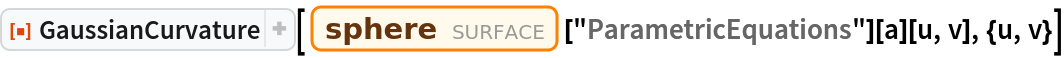Out=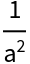### Scope (3)

Plot an astroidal ellipsoid:

 In:=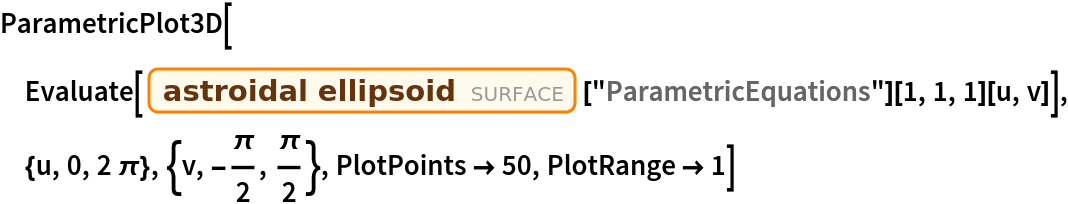Out=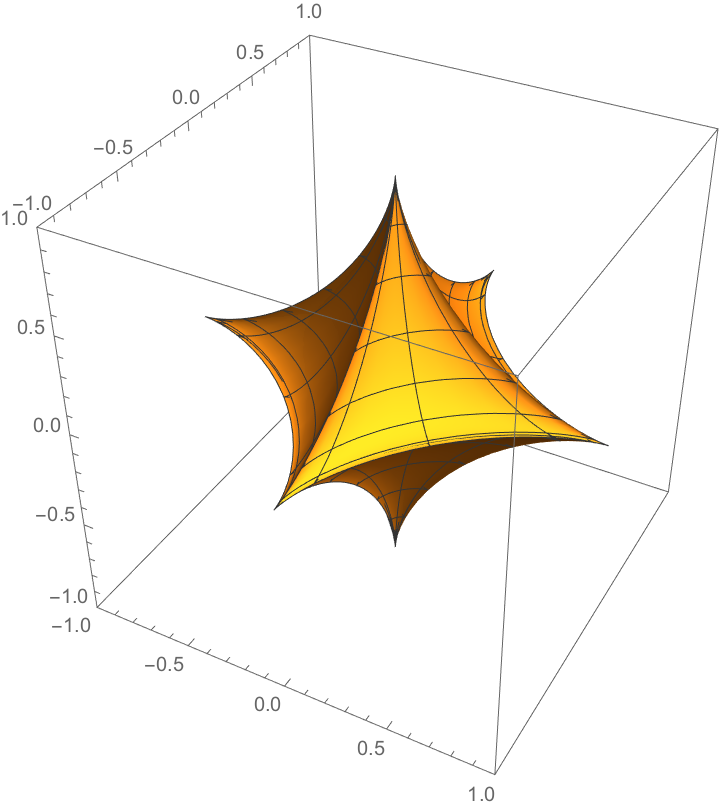Now compute its Gaussian curvature:

 In:=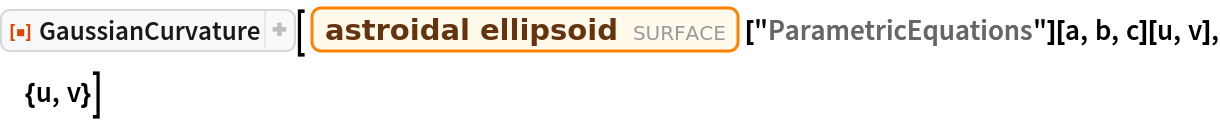Out=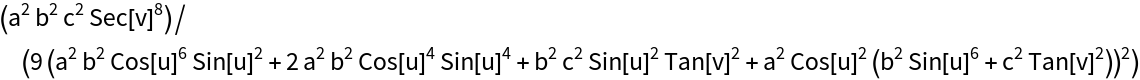Plot the Gaussian curvature:

 In:=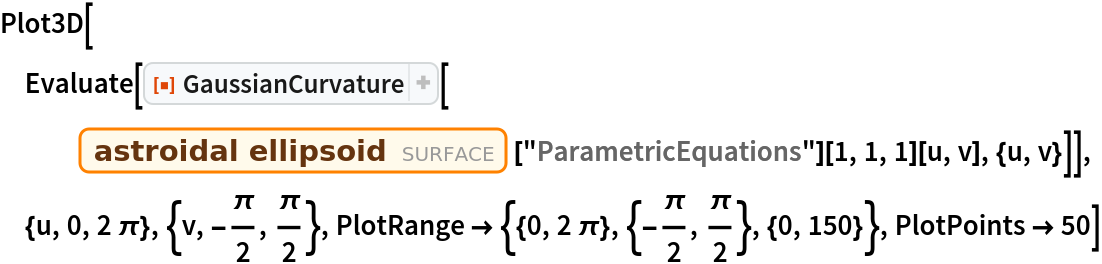Out=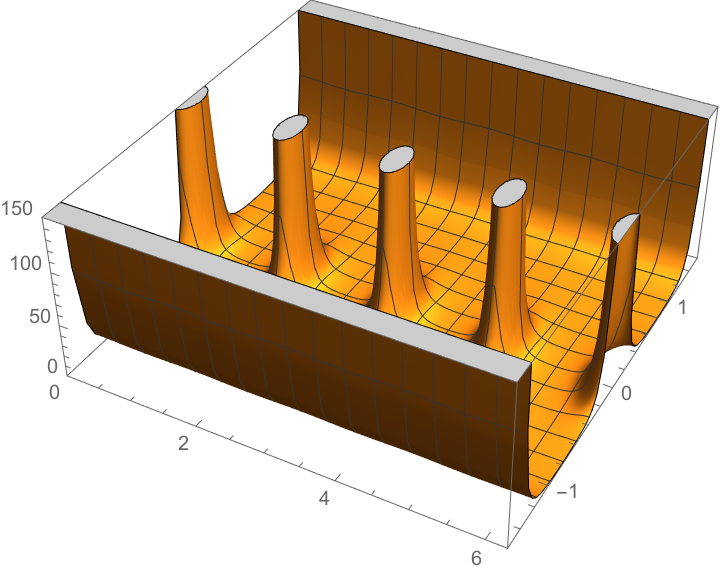Define a Möbius strip:

 In:=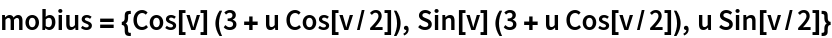Out=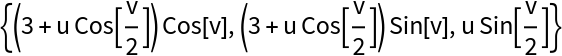Compute the Gaussian curvature:

 In:=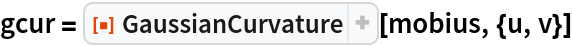Out=Plot the Gaussian curvature:

 In:=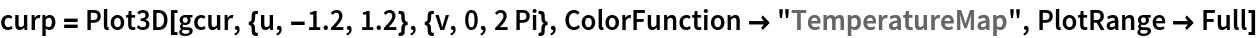Out=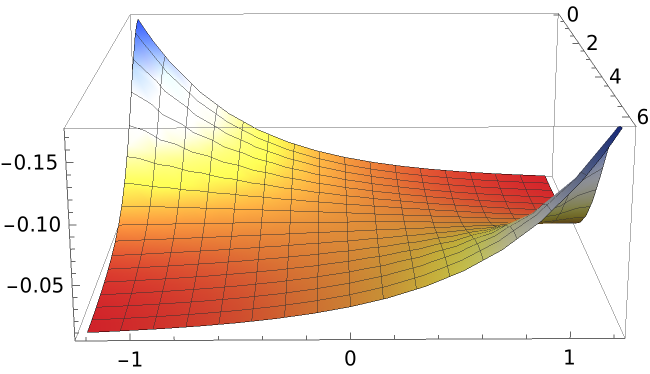Plot the Möbius strip colored according to its Gaussian curvature:

 In:=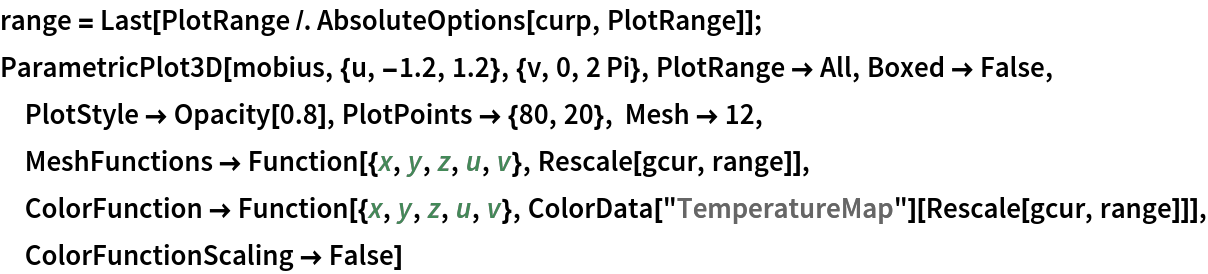Out=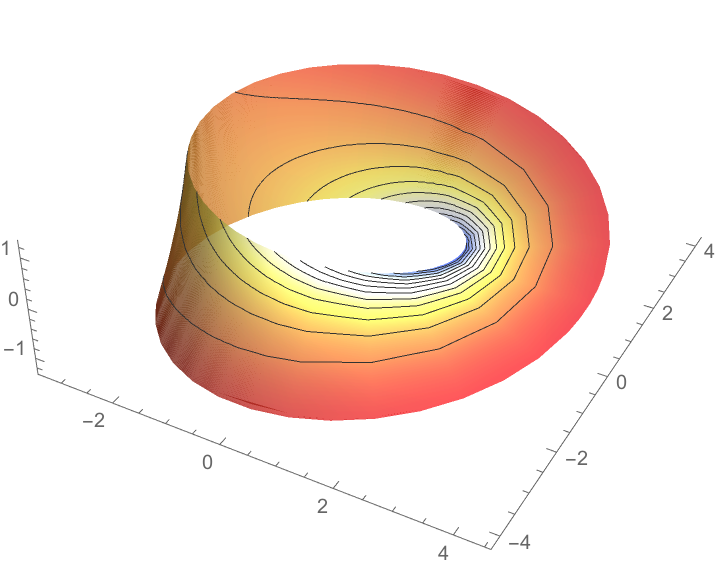Here is an implicit equation for a surface:

 In:=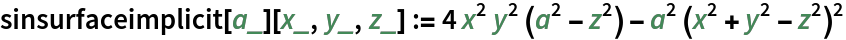Plot it:

 In:=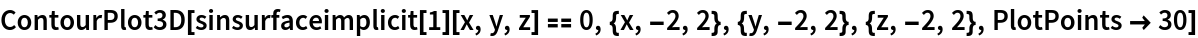Out=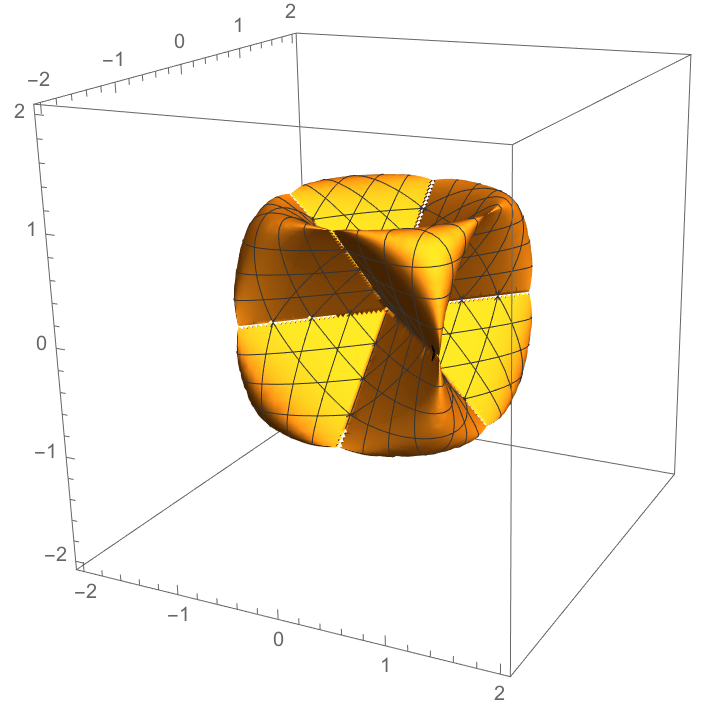Compute its Gaussian curvature:

 In:=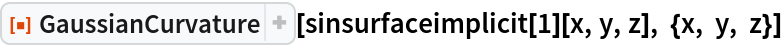Out=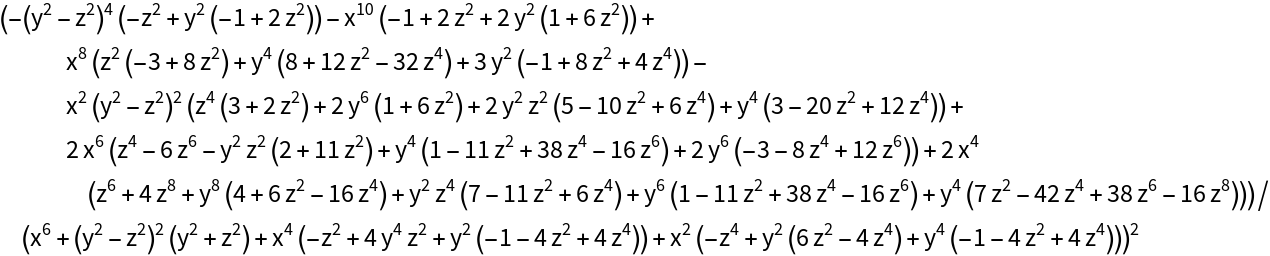### Properties and Relations (3)

The curvature can be obtained for named surfaces using entities:

 In:=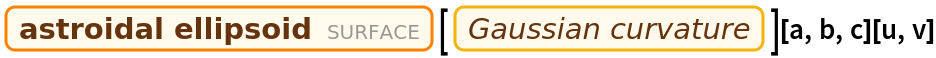Out=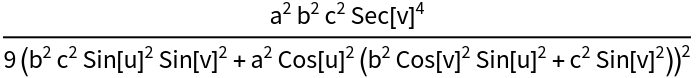Here is the result returned by GaussianCurvature:

 In:=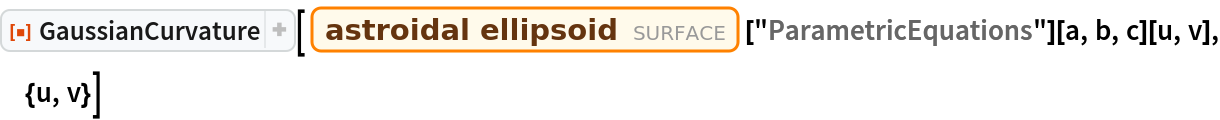Out=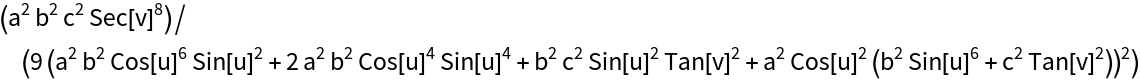The expressions seem to be different, but they are equal:

 In:=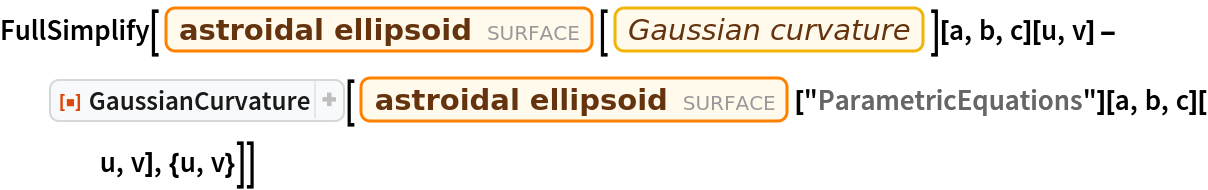Out=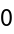Enrique Zeleny

## Version History

• 2.1.0 – 04 January 2021
• 2.0.0 – 01 April 2020
• 1.0.0 – 13 February 2020